CBSE Test Paper 01

1. E Divides AB in the ratio 1 : 3 and also, F divides AC in the ratio 1 : 3. EF = 2.8cm, Find BC
1. 11.2cm
2. 11cm
3. 11.5cm
4. 12cm
2. The figure formed by joining the mid-points of the sides of a quadrilateral ABCD, taken in order, is a square only if

1. ABCD is a Rhombus

2. Diagonals of ABCD are equal and perpendicular

3. Diagonals of ABCD are perpendicular

4. Diagonals of ABCD are equal

3. In fig if DE = 8 cm, DE $\parallel$ BC and D is the mid-Point of AB, then the true statement is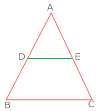1. E is the mid-Point of AC

2. AB = BC

3. DE = BC

4. DE and BC meet at some point if we extend both of them indefinitely.

4. In fig D is mid-point of AB and DE $\parallel$ BC then AE is equal to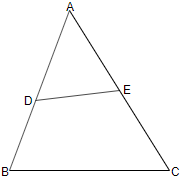2. DB

3. BC

4. EC

5. In a triangle ABC, P, Q and R are the mid-points of the sides BC, CA and AB respectively. If AC = 21cm, BC = 29cm and AB = 30cm, find the perimeter of the quadrilateral ARPQ?

1.   20cm

2.  80cm

3.  51cm

4.   52cm

6. Fill in the blanks:

If in a parallelogram its diagonals bisect each other at right angles and are equal, then it is a ________.

7. Fill in the blanks:

If the two non-parallel sides of a trapezium are equal, then it is called an ________  trapezium.

8. ABCD is a parallelogram. If its diagonals are equal, then find the value of $\mathrm{\angle }$ABC.

9. In a parallelogram PQRS, if $\mathrm{\angle }$P = (3x - 5)o and $\mathrm{\angle }$Q = (2x +15)o. Find the value of x.

10. In figure, $\mathrm{\angle }$B < $\mathrm{\angle }$A and$\mathrm{\angle }$C < $\mathrm{\angle }$D. Show that AD < BC.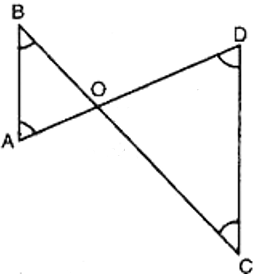11. If the angles of a triangle are in the ratio 1 : 2 : 3, determine three angles.

12. In $\mathrm{△}$ABC, if $\mathrm{\angle }$A = 120o and AB = AC. Find $\mathrm{\angle }$B and $\mathrm{\angle }$C.

13. Show that the line segments joining the mid-points of the opposite sides of a quadrilateral bisect each other.

14. PQ and RS are two equal and parallel line-segments. Any point M not lying on PQ or RS is joined to Q and S and lines through P parallel to QM and through R parallel to SM meet at N. Prove that line segments MN and PQ are equal and parallel to each other.

15. Show that the line segments joining the mid-points of opposite sides of a quadrilateral bisect each other.

CBSE Test Paper 01

Solution

1. (a) 11.2cm
Explanation:
Let AE = x and EB = 3x , AF = y and FC = 3y.
EF = 2.8 cm
AE + AF = 2.8 implies x + y = 2.8
BC = CF + FA + AE + EB
= 3y + y + x + 3x
= 4 ( x + y ) = 4 ( 2.8 ) = 11.2 cm
2. (b) Diagonals of ABCD are equal and perpendicular
Explanation: A quadrilateral formed by joining the mid points of a square is a square. So, ABCD is a square. In Square, diagonals are equal and perpendicular.
3. (a) E is the mid-Point of AC
Explanation: By the converse of Mid Point Theorem, which states that," If a line segment is drawn passing through the midpoint of any one side of a triangle and parallel to another side, then the line segment bisects the remaining third side.
4. (d) EC
Explanation: By midpoint theorem of a triangle E is the midpoint of AC, hence AE = EC
5. (c) 51cm
Explanation:
Given:
A B C is a triangle .

P Q R are the mid points of sides BC , CA nad AB .

AC = 21 cm

BC = 29 cm

AB = 30 cm

To find :

Q is the mid point of AC

P is the mid point of BC

QP is parallel to AB

QP = half of AB ( according to mid point theorem )

AB = 30 cm , QP = 15 CM ( QP is half of BA ) ( proved above )

R is the mid point of side AB

QP is also parallel to AR ( half of side AB )

PR is parallel to AC

PR = half of AC (according to mid point theorem )

AC = 21 cm , PR = 10.5 cm ( PR is half of AC ) ( proved above )

PR is parallel to AQ ( AQ is half of AC )

Since , in quadrilateral ARQP both the opposite sides are parallel it is a parallelogram.

Therefore , ARQP is a parallelogram .

WE know that

In parallelogram , opp sides are equal .

Therefore ,

PR = AQ = 10.5 cm

QP = AR = 15 cm

10.5 cm + 10.5 cm + 15 cm + 15 cm = 51 cm .

Therefore the perimeter of quadrilateral ARQP = 51 cm .

6. Square

7. isosceles

8. As diagonals of the parallelogram ABCD are equal, hence it is a rectangle. We know that, each angle of the rectangle is 90° .
$\therefore$ $\mathrm{\angle }$ABC = 90o

9. $\mathrm{\angle }P+\mathrm{\angle }Q={180}^{\circ }$ (Angles on the same side of a transversal are supplementary)

5x + 10 = 180o
$⇒$ 5x + 170o $⇒$ x = 34o

10. In $\mathrm{△}$AOB,
$\mathrm{\angle }$B <$\mathrm{\angle }$ A [Given]
$⇒$OA < OB ...(i) [Side opposite to greater angle is longer]
In$\mathrm{\Delta }$ COD,
$\mathrm{\angle }$C < $\mathrm{\angle }$D [Given]
$⇒$OD < OC ...(ii) [Side opposite to greater angle is longer]
OA + OD < OB + OC
$⇒$AD < BC

11. Let the angles be x, 2x, 3x
$\therefore$ x + 2x + 3x = 180o (sum of all angles of a triangle)
$⇒$ 6x = 180o
$⇒$ x = 30o
Since x = 30o
2x = 2 $×$ 30o = 60o
3x = 3 $×$ 30o = 90o
$\therefore$ angles are 30o, 60o, 90o

12.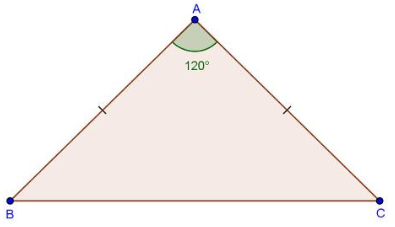In $\mathrm{△}$ABC
$\because$ AB = AC
$⇒\mathrm{\angle }B=\mathrm{\angle }C$ = x [Angle opposite to equal sides are equal]
Now in $\mathrm{△}$ABC,
$\mathrm{\angle }A+\mathrm{\angle }B+\mathrm{\angle }C={180}^{\circ }$
$⇒$120$\circ$+ x + x = 180$\circ$
$⇒$ 2x = 180$\circ$ - 120$\circ$
$⇒2x={60}^{\circ }$
$⇒$ x = 30$\circ$
$⇒\mathrm{\angle }B=\mathrm{\angle }C={30}^{\circ }$

13. ABCD is a quadrilateral P, Q, R and S are the mid-points of the sides DC, CB, BA and AD respectively.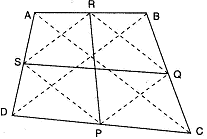To prove : PR and QS bisect each other.
Construction : Join PQ, QR, RS, SP, AC and BD.
Proof : In $\mathrm{△}$ABC,
As R and Q are the mid-points of AB and BC respectively.
$\therefore$ RQ || AC and RQ = $\frac{1}{2}$ AC
Similarly, we can show that
PS || AC and PS = $\frac{1}{2}$ AC
$\therefore$ RQ || PS and RQ = PS.
Thus a pair of opposite sides of a quadrilateral PQRS are parallel and equal.
$\therefore$ PQRS is a parallelogram.
Since the diagonals of a parallelogram bisect each other.
$\therefore$ PR and QS bisect each other.

14. Given: PQ = RS, PQ $\parallel$ RS, PN $\parallel$ QM, RN $\parallel$ MS
To prove: MN = PQ, MN $\parallel$ PQ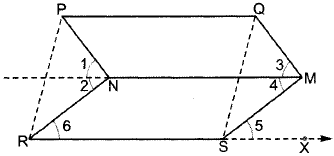Proof: Since PQ = RS and PQ $\parallel$ RS, therefore PQSR is a parallelogram.
$⇒$ PR = QS, PR $\parallel$ QS
Since PN $\parallel$ QM and MN is the transversal, we have
$\mathrm{\angle }$1 = $\mathrm{\angle }$3   (Corresponding angles) ......................................(i)
Similarly, RN $\parallel$ MS, we have
$\mathrm{\angle }$2 = $\mathrm{\angle }$4                         ...........................................(ii)
Adding (i) and (ii), we obtain
$\mathrm{\angle }$1 + $\mathrm{\angle }$2 = $\mathrm{\angle }$3 + $\mathrm{\angle }$4 i.e., $\mathrm{\angle }$PNR - $\mathrm{\angle }$QMS
Again, $\mathrm{\angle }$PRS = $\mathrm{\angle }$QSX ........(Corresponding angles as PR $\parallel$ QS)
and $\mathrm{\angle }$6 = $\mathrm{\angle }$5 .......................(Corresponding angles as RN $\parallel$ SM)
Subtracting the above two equations, we get
$\mathrm{\angle }$PRS - $\mathrm{\angle }$6 = $\mathrm{\angle }$QSX - $\mathrm{\angle }$5 i.e., $\mathrm{\angle }$PRN = $\mathrm{\angle }$QSM
Now, in $\mathrm{\angle }$PNR and $\mathrm{\angle }$QMS,
PR - QS (Opp. sides of $\parallel$gm)
$\mathrm{\angle }$PNR = $\mathrm{\angle }$QMS (Proved above)
$\mathrm{\angle }$PRN = $\mathrm{\angle }$QSM
Therefore, By AAS congruence criterion, we have

$\mathrm{△}$PNR = $\mathrm{△}$QMS
$⇒$ PN = QM (CPCT)
Also, PN $\parallel$ QM (Given)
Therefore, PNMQ is a parallelogram.
$⇒$ PQ $\parallel$ MN and PQ = MN.

15. Given: A quadrilateral ABCD in which EG and FH are the line-segments joining the mid-points of opposite sides of a quadrilateral.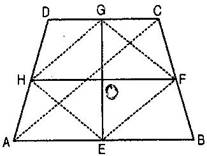To prove: EG and FH bisect each other.
Construction: Join AC, EF, FG, GH and HE.
Proof: In ABC, E and F are the mid-points of respective sides AB and BC.
$\therefore$ EF || AC and EF = $\frac{1}{2}$ AC ……….(i)
$\therefore$HG || AC and HG = $\frac{1}{2}$ AC ……….(ii)
$\therefore$EFGH is a parallelogram.